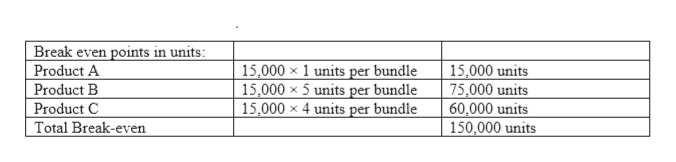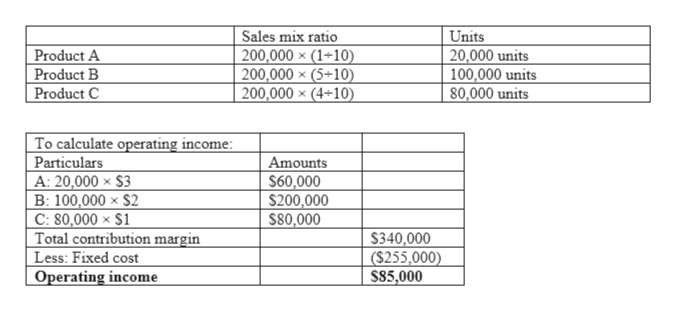# Sales mix, three products. The Ronowski Company has three product lines of belts—A, B, and C— with contribution margins of \$3, \$2, and \$1, respectively. The president foresees sales of 200,000 units in the coming period, consisting of 20,000 units of A, 100,000 units of B, and 80,000 units of C. The company’s fixed costs for the period are \$255,000.What is the company’s breakeven point in units, assuming that the given sales mix is maintained?If the sales mix is maintained, what is the total contribution margin when 200,000 units are sold? What is the operating income?What would operating income be if 20,000 units of A, 80,000 units of B, and 100,000 units of C were sold? What is the new breakeven point in units if these relationships persist in the next period?

Question
58 views

Sales mix, three products. The Ronowski Company has three product lines of belts—A, B, and C— with contribution margins of \$3, \$2, and \$1, respectively. The president foresees sales of 200,000 units in the coming period, consisting of 20,000 units of A, 100,000 units of B, and 80,000 units of C. The company’s fixed costs for the period are \$255,000.

1. What is the company’s breakeven point in units, assuming that the given sales mix is maintained?
2. If the sales mix is maintained, what is the total contribution margin when 200,000 units are sold? What is the operating income?
3. What would operating income be if 20,000 units of A, 80,000 units of B, and 100,000 units of C were sold? What is the new breakeven point in units if these relationships persist in the next period?
check_circle

Step 1

Cost-volume profit analysis is a tool that helps in decision making by establishing a relationship between volume, price, variable cost, fixed cost and profit.

Step 2
1. To calculate break even in units:

Ratio of sales mix= A: B: C= 20,000:100,000: 80,000

= 1:5:4

Contribution margin of the bundle= 1 ×\$3 + 5× \$2 + 4× \$1

= \$17

Break-even point in bundles= Fixed cost ÷ Contribution margin per bundle

= \$255,000 ÷ \$17

= 15,000 bundleshelp_outlineImage TranscriptioncloseBreak even points in units: 15,000 x 1 units per bundle 15,000 × 5 units per bundle 15,000 x 4 units per bundle 15,000 units 75,000 units 60,000 units 150,000 units Product A Product B Product C Total Break-even fullscreen
Step 3
1. If the no. of units is sold are \$...help_outlineImage TranscriptioncloseUnits 20,000 units 100,000 units 80,000 units Sales mix ratio 200,000 × (1+10) 200,000 × (5+10) 200,000 × (4-10) Product A Product B Product C To calculate operating income: Particulars A: 20,000 × \$3 B: 100,000 × \$2 C: 80,000 × \$1 Total contribution margin Less: Fixed cost Operating income Amounts S60,000 \$200,000 S80,000 \$340,000 (\$255,000) S85,000 fullscreen

### Want to see the full answer?

See Solution

#### Want to see this answer and more?

Solutions are written by subject experts who are available 24/7. Questions are typically answered within 1 hour.*

See Solution
*Response times may vary by subject and question.
Tagged in